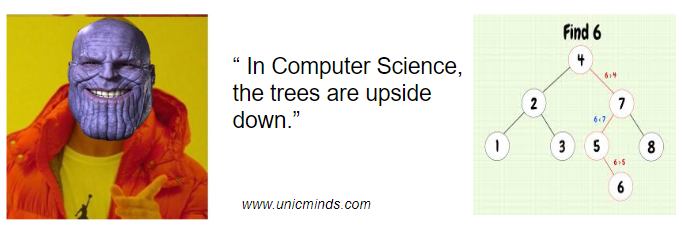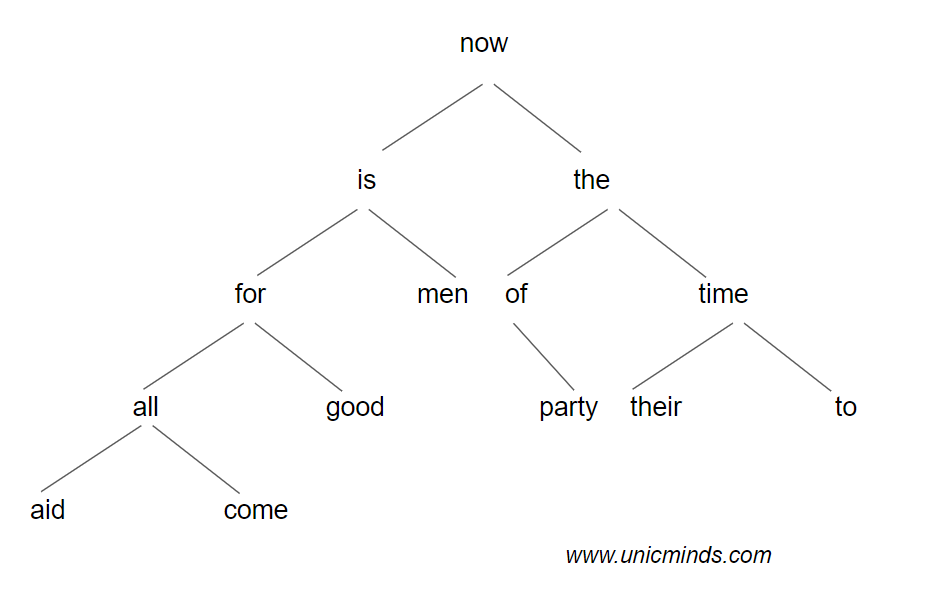Unicminds# Binary Trees of Computer Science – A bit twisted!

The above picture shows how normal trees are in nature. Well, trees in computer science are inverted.### Introduction to Binary Trees

A binary tree is a way in which data is stored and organized so that when you want to find something it is easier and faster and takes up less time and space. When you search something in your computer or when you’re compressing files in your computer, it does its job in a jiffy (or rather almost a jiffy). How does the computer do these things so fast? How does it know where a particular file is: Well, the short and simple answer is, the computer uses binary trees to organize itself.

Let’s say you want to write a simple program that will read an input line and count the number of occurrences (frequency) of a particular word in the sentence. Let’s take the popular sentence: “Now is the time for all good men to come to the aid of the party”.

The program would take too long. For one linear loop to run from 1 to n, it would take T(n) processing time. Since you need to have two linear loops nested one under another, this program would take T(n^2) processing time to complete. That will be too long.

### Reducing Complexity of Algorithms

One should keep in mind that a single loop increases time linearly and a nested loop increases time polynomially. So, to reduce time, one has to keep the set of words sorted all the time by keeping each word in its proper position all the time as it arrives while parsing the sentence. This shouldn’t be done by shifting the linear array (which will again take too long time). The solution is to use a data (organizing) structure called Binary Search Tree.

The tree is maintained such that at any node the left sub-tree contains only words that are less than the word at the node and the right sub-tree contains only words that are greater than the word at the node. See below, all left side sub-tree has alphabetically lesser words than the node and the right sub-tree has alphabetically greater words than the node.

There are many data structures in computer-science like arrays, lists, linked-lists, stacks, queues, etc. and these are all linear data structures whereas binary trees are non-linear data structures that have certain advantages over linear structures.If you see, when you’ve to search something in this tree, you don’t navigate linearly through all the nodes like how you do in an array. You cut through the tree and search only some nodes of the tree. Therefore, the processing time would be less in binary trees – T(n/2) or in the worst case T(n). Now, think about how you can use this in writing code for a “Find the robber in your apartment” game.

There are different types of trees implemented in computer science and these data structures are one of the key paradigm designs of computers in the way a lot of algorithms are written and how computers do their daily work.

Hope this is useful, thank you.

BOOK A FREE TRIAL